Download PresentationThe table below shows the distance ran by a cross-country runner.

# The table below shows the distance ran by a cross-country runner. - PowerPoint PPT Presentation

The table below shows the distance ran by a cross-country runner. Which graph would be most appropriate to show the data and why? Line graph, bar graph, line plot, or circle graph?. 2. Name an ordered pair that is inside both the circle and the triangle.I am the owner, or an agent authorized to act on behalf of the owner, of the copyrighted work described.
Download Presentation## The table below shows the distance ran by a cross-country runner.

An Image/Link below is provided (as is) to download presentation

Download Policy: Content on the Website is provided to you AS IS for your information and personal use and may not be sold / licensed / shared on other websites without getting consent from its author.While downloading, if for some reason you are not able to download a presentation, the publisher may have deleted the file from their server.

- - - - - - - - - - - - - - - - - - - - - - - - - - E N D - - - - - - - - - - - - - - - - - - - - - - - - - -
Presentation Transcript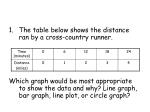Which graph would be most appropriate to show the data and why? Line graph, bar graph, line plot, or circle graph?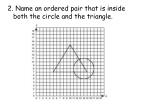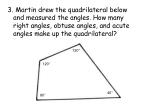3. Martin drew the quadrilateral below and measured the angles. How many right angles, obtuse angles, and acute angles make up the quadrilateral?

120°

120°

40°

80°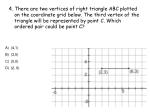4. There are two vertices of right triangle ABC plotted on the coordinate grid below. The third vertex of the triangle will be represented by point C. Which ordered pair could be point C?
• (4,1)
• (3,5)
• (0,0)
• (2, 6)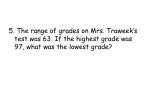5. The range of grades on Mrs. Traweek’s test was 63. If the highest grade was 97, what was the lowest grade?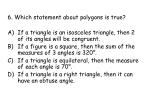6. Which statement about polygons is true?
• If a triangle is an isosceles triangle, then 2 of its angles will be congruent.
• If a figure is a square, then the sum of the measures of 3 angles is 320°.
• If a triangle is equilateral, then the measure of each angle is 70°.
• If a triangle is a right triangle, then it can have an obtuse angle.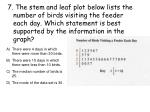7. The stem and leaf plot below lists the number of birds visiting the feeder each day. Which statement is best supported by the information in the graph?
• There were 4 days in which there were more than 30 birds.
• There were 15 days in which there were less than 10 birds.
• The median number of birds is 25.
• The mode of the data set is 30.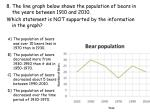8. The line graph below shows the population of bears in the years between 1910 and 2010.

Which statement is NOT supported by the information in the graph?

• The population of bears was over 10 bears less in 1970 than in 1930.
• The population of bears decreased more from 1930-1950 than it did in 1950-1970.
• The population of bears decreased about 5 bears between 1990-2010.
• The population of bears decreased over the time period of 1930-1970.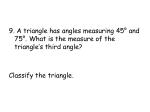9. A triangle has angles measuring 45° and 75°. What is the measure of the triangle’s third angle?

Classify the triangle.11. The circle graph below shows the percent of students at Doerre who walk, bike, car ride, or bus ride to school each day.

If there are 40 students who walk, how many students ride in a car?12. Mrs. Williams took a trip to Chicago and the temperature was below 0°. According to the thermometer, what is the temperature?13. The clock shows the time 3:30, creating an acute angle. When the clock shows the time 3:45, what type of angle will it create?15. The graph below shows the number of people who prefer different types of movies. What is the mean number for the 5 movie types?16. On Valentine’s Day, Leigh bought 15 carnations. She gave away 9 to girls. Then, she gave away 2 to boys. At the end of the day, she received 4 carnations from others. Write a number sentence to represent this situation.17. Which statement is NOT supported by the graph?
• There are more males than females.
• There are approximately 3 more Roberts members than Brown members.
• There are approximately 3 more females in O’Hara than in Roberts.
• There are approximately 60 people at the reunion.18. The bar graph below shows the number of turkeys at Kroger that fit into different weight groups.

Which statement is best supports by the information in the graph?

• The 21 pound turkey group had twice as many turkeys as the 20 pound group.
• The 24 pound turkey group had 4 times as many turkeys as the 22 pound group.
• The 24 pound turkey group had more turkeys than the 25 pound and 26 pound groups combined.
• The 28 pound turkey group had half as many turkeys as the 21 pound turkey group.19. The table below shows the weight of 15 watermelons. Create a histogram using the information in the table.